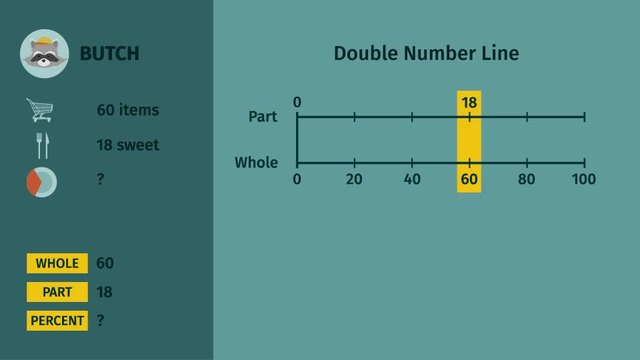# Visualizing the Percent of a QuantityRating

Be the first to give a rating!

The authorsSarah Betack

## Basics on the topicVisualizing the Percent of a Quantity

After this lesson, you will be able to solve percent problems using models or diagrams.

The lesson begins by teaching you that the three components in solving percent problems are the whole, part, and percent. It leads you to learn that models like tape diagrams, 10x10 grids, or tape diagrams can be used to visually solve a percent problem. It concludes by noting that if you have two of the three components then you can always find the third.

Learn about visualizing the percent of a quantity by helping the raccoon brothers sort out their groceries.

This video includes key concepts, notation, and vocabulary such as percent (part per hundred); whole (the total amount of a certain quantity); and part (an amount of the whole).

Before watching this video, you should already be familiar with ratios, proportions, multiplication and division, tape diagram, and the double number line.

After watching this video, you will be prepared to learn how to use ratios to convert measurement units; manipulate and transform units appropriately when multiplying or dividing quantities.

Common Core Standard(s) in focus: 6.RP.A.3.C A video intended for math students in the 6th grade Recommended for students who are 11 - 12 years old